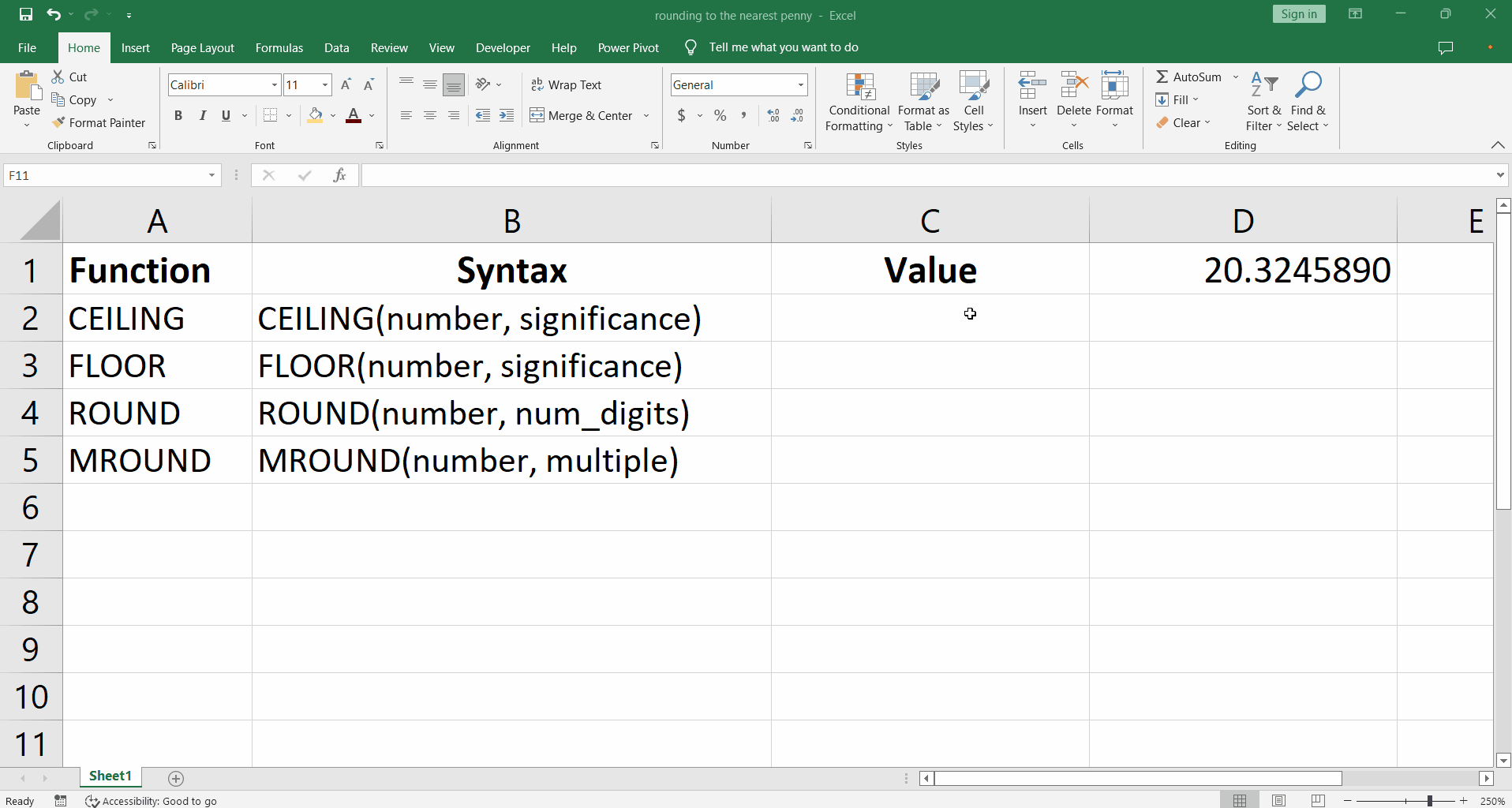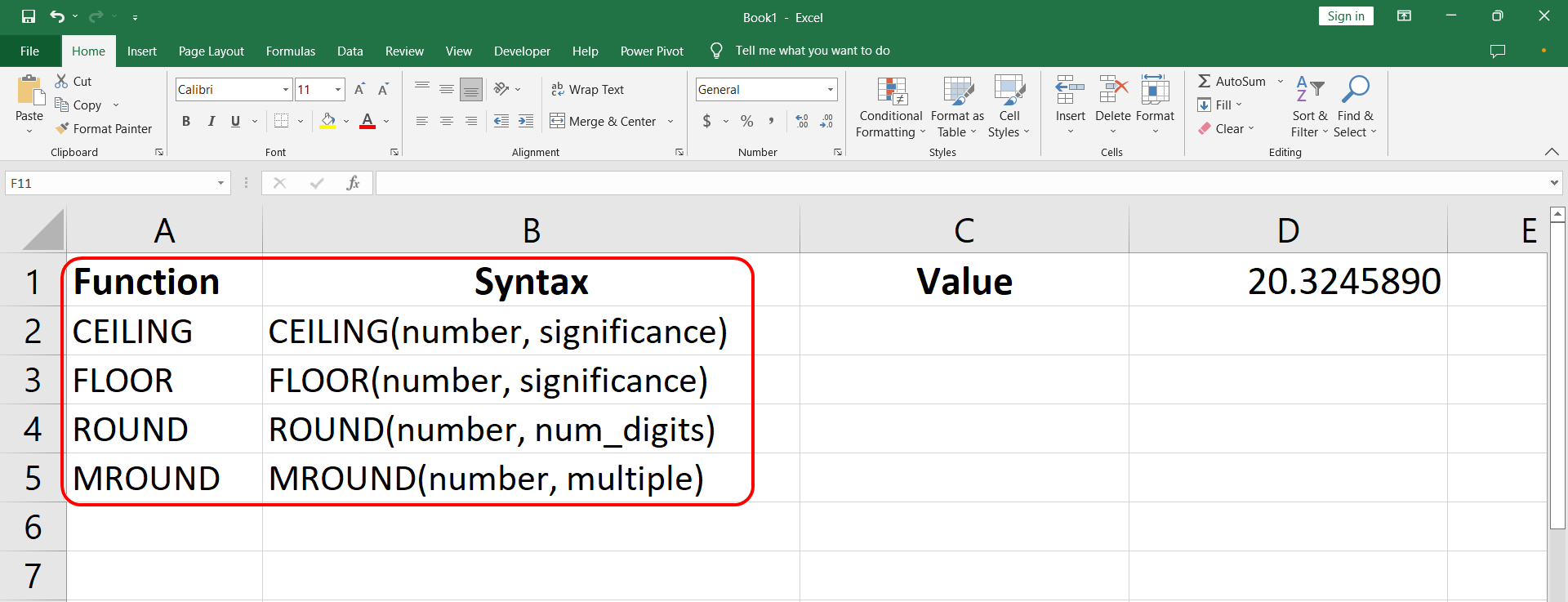# How to round to the nearest penny in Excel

Suppose we have a value we want to round off to the nearest penny as shown above.While working with currency we might want to round off a value to a desired significant number. One such requirement is to round off to the nearest penny which is of course a hundredth of a pound. This can be done using four different functions in excel.

CEILING function
– FLOOR function
– ROUND function
– MROUND function

CEILING and FLOOR functions round upwards and downwards respectively. ROUND considers if the next significant digit is greater than or less than 5 and rounds upward or downward accordingly. MROUND considers the nearest multiple to which the number must be rounded to.

### Step 1 – Understanding syntax of functions– The syntax for each of the functions involves an attribute for the original value and the number of significant digits to which the number is to be rounded to.

– The syntax for all four functions is shown in the image below.

– In order to round the value in D1 to the nearest penny, the first attribute will take D1 as reference for the value.

– In case of CEILING and FLOOR functions, 0.01 will go in as significance, because we wish to round off to the hundredth part of a pound.

– For the ROUND function 2 is the num_digits. Which tells the function to keep two digits after the decimal point.

– Similarly, for MROUND 0.01 will go as the multiple. Here 0.01 is used again to tell the function that we wish to keep two digits after the decimal point.

### Step 2 – Implement the functions– To implement the function type the following text =CEILING(D1, 0.01) in the cell C1.

– Similarly type all the functions starting with equal sign ‘=’ followed by the function names, and the attributes D1 and 0.01 between the parentheses. The implementation can be seen as follows in the above picture.